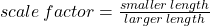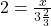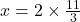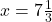## Yasmin designed a square table using a scale drawing. The actual length of each side was 4 feet and the scale facto was 1 inch : 2 fee

Question

Yasmin designed a square table using a scale drawing. The actual length of each side was 4 feet and the scale facto
was 1 inch : 2 feet. Choose the figure with the correct side length if Yasmin changed the scale factor to 1 inch: 3 2/3 feet.

A. X = 2ft

B. X = 6 2/3

C. X = 7 1/3

D. X = 14 2/3

Note: please explain how to get the answer because I kept getting D as my answer. Is there a mistake I made? And please do not answer with links.

in progress 0
5 months 2021-09-05T04:45:13+00:00 2 Answers 0 views 0

## Answers ( )

It is x = 7 1/3

Step-by-step explanation:

2 feet is being used in the scale factor and 2 x 3 2/3 is 7 1/3.

Please mark as brainliest

The scale factor is a measure for similar figures, who look the same but have different scales or measures. Suppose, two circle looks similar but they could have varying radii. The scale factor states the scale by which a figure is bigger or smaller than the original figure.

we have:let the length be x.

we haveso.

C .is your answer